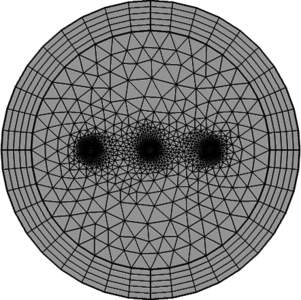# Technical Papers and Presentations

## Forces on Parallel Three-Phase AC-Conductors During A Phase to Ground Fault

D. Edström, M. Ögren, N. Lavesson
Örebro University, Sweden
ABB Corporate research, Sweden
Published in 2019

We consider the problem of calculating forces on high current solid conductors, as is present e.g. in substations. This is of importance for the design of the station, in particular for the conductor geometry and mechanical support. The full calculation includes a treatment of the skin effect due to the alternating-currents and the relatively large cross sections of the conductors. Forces on each of the conductors are calculated and studied as function of the short circuit current and the geometry for a phase to ground fault.

We have used COMSOL Multiphysics® 5.4 with the AC/DC Module. Focus here are on 2D-geometries, assuming long straight conductors to penetrate the 2D plane perpendicular, although also some 3D simulations have been carried out to compare with the 2D results and as initial tests of more complicated conductor geometries. Assuming harmonic alternating-currents, frequency studies (mainly for 50 Hz) of the forces have been carried out. In addition, the inverse discrete Fourier transform have been applied to create time-series to compare to full time dependent simulations. After such numerical tests were satisfactory, we introduced more realistic models for the currents during a phase to ground short circuit fault and carried out full time dependent simulations of the forces. Forces on each part of the conductors can be calculated from the Lorentz force. To obtain the total forces on each conductor, surface integrals were used. Alternatively, the forces can be calculated via the Maxwell stress tensor with the conductor’s circumference defining the path for the corresponding line integral in Greens’ formula.

As a first example, we have investigated the skin effect in one cylindrical AC-conductor for various frequencies and compared the numerical results for the current density and resistance to well-known analytic models . Secondly, we reproduced the well-known case of forces between two parallel DC conductors , which up until 19th of May 2019 have been the basis for defining the SI-unit ampere .

After those initial tests we set up a geometry, containing parameters for all positions and distances to be included in a COMSOL® App, to treat three AC conductors in parallel. The obtained time-series of the forces for stationary AC were sinusoidal as expected. Introducing more realistic short circuit currents resulted in complicated time-series for the forces. Those in turn will form important input to mechanical engineers for the design of e.g. substations.

We initially show that a COMSOL® implementation using the AC/DC Module reproduce a well-known example for the skin effect. After successfully comparing the outcome from frequency studies and time dependent studies for stationary AC, we are confident in the results for forces calculated from a time dependent study for a non-trivial short circuit current during a phase to ground fault. In future work we will create a simulation App to be useful within the electric power industry.

 IEEE Standard C37.12-2008 - IEEE Guide for Bus Design in Air Insulated Substations
 Jordan, Edward Conrad (1968), Electromagnetic Waves and Radiating Systems, Prentice Hall, ISBN 978-0-13-249995-8.
 www.comsol.se/model/electromagnetic-forces-on-parallel-current-carrying-wires-131
 https://en.wikipedia.org/wiki/2019_redefinition_of_SI_base_units#Ampere